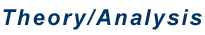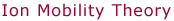Experimental Collision Cross Sections     The reduced mobility K0 of an ion drifting through a buffer gas, discussed in the previous section, can be related to its collision cross section using kinetic theory.In this expression, e is the charge on the ion, N0 the buffer gas number density at P0 and T0, μ the reduced mass of the buffer gas and ion, T the effective temperature, and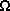the momentum transfer collision integral. Thus, by measuring K0 we obtain a value for, an experimental "cross section".     Kinetic theory indicates that the quantityis a momentum transfer collision integral. It is generally very difficult to calculate theoretically, unless it is calculated for a rigid sphere, in which caseis equal to the projection cross section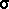. For a general geometrical shape the projection cross section, which is fairly straight forward to calculate for any shape, is only an approximation for the collision integral.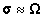However, for similar shapes, differences betweenandare similar and can be corrected for empirically. Differences betweenandare largest (up to 20%) for exotic geometries with large concave surfaces like bowls. (See e.g. Shvartsburg, A. A.; Jarrold, M. F. Chem. Phys. Lett. 1996, 261, 86-91 and Shvartsburg, A. A.; Schatz, G. C.; Jarrold, M. F. J. Chem. Phys. 1998, 108, 2416-2423.) See also: "Theory/Analysis: Theoretical Collision Cross Sections"